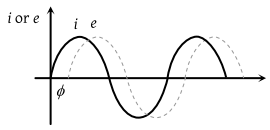NEET Physics Alternating Current Questions Solved

When an ac source of e.m.f. $e={E}_{0}\mathrm{sin}\left(100\text{\hspace{0.17em}}t\right)$ is connected across a circuit, the phase difference between the e.m.f. e and the current i in the circuit is observed to be &pi:/4, as shown in the diagram. If the circuit consists possibly only of RC or LC in series, find the relationship between the two elements(1) $R=1k\Omega ,\text{\hspace{0.17em}}C=10\mu F$

(2) $R=1k\Omega ,\text{\hspace{0.17em}}C=1\mu F$

(3) $R=1k\Omega ,\text{\hspace{0.17em}}L=10H$

(4) $R=1k\Omega ,\text{\hspace{0.17em}}L=1H$

Explanation is a part of a Paid Course. To view Explanation Please buy the course.

Difficulty Level: